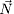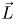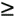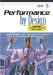# 14.8 Exercises

1. Consider the CS model discussed in Example 14.2. What would be the impact of using a 10-Mbps Ethernet instead of the original 100-Mbps Ethernet. Assume that the 10-Mbps Ethernet has a slot duration S = 51.2 msec and a maximum packet length, Lp, including header and payload of 1518 bytes.

2. In the the two-tier client-server architecture of Example 14.4, the assumed speed of the LAN is 10 Mbps. What is the response time of the three classes of requests if the LAN were replaced by a faster one, with a bandwidth of 100 Mbps.

3. Consider Example 14.4. Assume that the average number of running processes is to be doubled. The proportion of processes that submit requests of each type (trivial, average, and complex) is kept constant. Thus, the percentage of requests of each type received by the server is unchanged. Compute the new values of the response time and throughput. Make and justify a recommendation to guarantee that the average transaction response time does not exceed 2 seconds.

4. An interactive computer system has M terminals used for a data-entry application. This application presents the user with a screen to be filled out before submitting it to the mainframe for processing. The computing system has one processor and two disks. The results of measurements taken during a 1 hour interval are shown in Table 14.7. Main memory at the mainframe is such that at most 5 transactions may be executed simultaneously. The company intends to redesign the user interface to increase the productivity at each terminal so that the average think time may be reduced to 60% of its original value. Also, the company expects that a change in the economy will boost its business so that more terminals will be needed. Under this new scenario, determine the maximum number of terminals, Mmax, the system will be able to handle before response time exceeds 3 sec. Plot a response time versus number of terminals curve. When the number of terminals is equal to Mmax, how much of the transaction response time is spent in the computer system, and how much is spent queuing for memory? Compute the processor and disk utilizations. Recommend and justify a system upgrade that will be able to handle 1.2 x Mmax terminals while keeping the average response time at 3 sec. Use MVA to answer the above questions. To take memory queuing into account, use MVA with load-dependent devices.

##### Table 14.7. Data for Exercise 14.4

Number of requests completed

11,808

Number of terminals (M)

100

Uprocessor

0.26

Udisk1

0.41

Udisk2

0.33

Avg. response time (sec)

0.47

5. Show that Eqs. (14.4.15) and (14.4.16) are the solution to Eq. (14.4.14). [Hint: Using Eq. (14.4.14), write Pi(1 |) as a function of Pi(0 |). Then write Pi(2 |) in terms of Pi(1 |). Since Pi(1 |) is already known to be a function of Pi(0 |, Pi(2 |) can be written as a function of Pi(0 |). Continue this process and observe what the general expression for Pi(j |) should be. Pi(0 |) can be computed by requiring that all probabilities sum to 1.]

6. Show that if device i is load-independent, Eqs. (14.5.24) and (14.5.25) reduce, (as expected) to Pi(j |) = (1 Ui)for j0.

7. ai(j) = ai(wi) for jwi prove Eqs. (14.5.26), (14.5.27), and 14.5.28). ( = ra[r+a (1 r)]/(1 r)2 for r < 1.)Performance by Design: Computer Capacity Planning By Example
ISBN: 0130906735
EAN: 2147483647
Year: 2003
Pages: 166